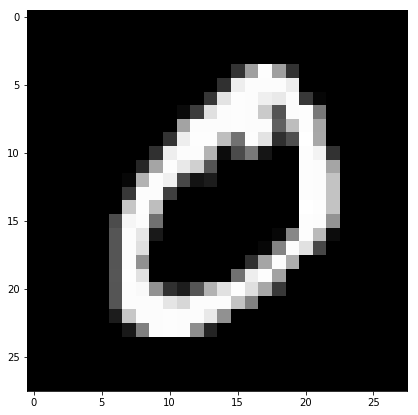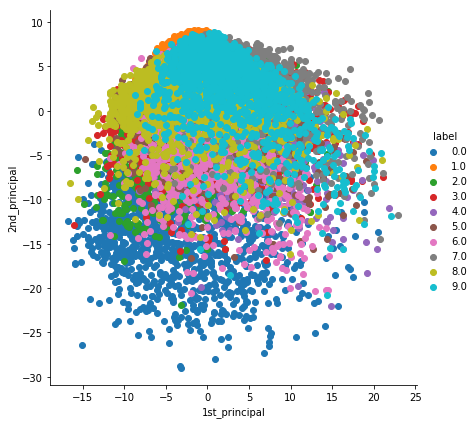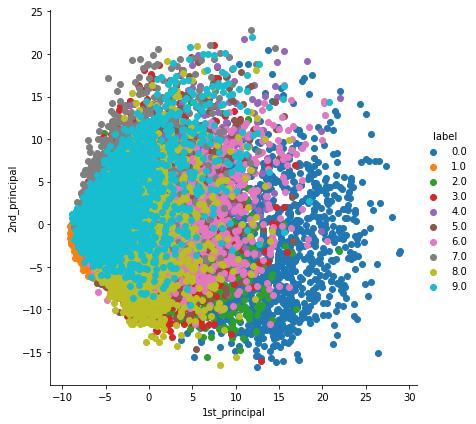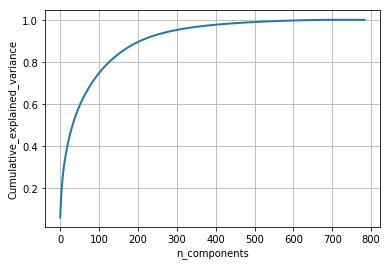### Load MNIST Data¶

In :
# Import python libabries.

import numpy as np
import pandas as pd
import matplotlib.pyplot as plt

#Import the data set

# print first five rows of d0.

# save the labels into a variable l.
l = d0['label']

# Drop the label feature and store the pixel data in d.
d = d0.drop("label",axis=1)


In :
d0.head(5)

Out:
label 1x1 1x2 1x3 1x4 1x5 1x6 1x7 1x8 1x9 ... 28x19 28x20 28x21 28x22 28x23 28x24 28x25 28x26 28x27 28x28
0 5 0 0 0 0 0 0 0 0 0 ... 0 0 0 0 0 0 0 0 0 0
1 0 0 0 0 0 0 0 0 0 0 ... 0 0 0 0 0 0 0 0 0 0
2 4 0 0 0 0 0 0 0 0 0 ... 0 0 0 0 0 0 0 0 0 0
3 1 0 0 0 0 0 0 0 0 0 ... 0 0 0 0 0 0 0 0 0 0
4 9 0 0 0 0 0 0 0 0 0 ... 0 0 0 0 0 0 0 0 0 0

5 rows × 785 columns

In :
print(d.shape)
print(l.shape)

(60000, 784)
(60000,)

In :
# display or plot a number.
plt.figure(figsize=(7,7))
idx = 1

# reshape from 1d to 2d pixel array
grid_data = d.iloc[idx].as_matrix().reshape(28,28)

# Visualize the grid data with the help of Matplotlib
plt.imshow(grid_data, interpolation = "none", cmap = "gray")
plt.show()

print(l[idx])

C:\ProgramData\Anaconda3\lib\site-packages\ipykernel_launcher.py:5: FutureWarning: Method .as_matrix will be removed in a future version. Use .values instead.
"""0


# 2D Visualization using PCA¶

In :
# Pick first 15K data-points to work on for time-effeciency.
# Excercise: Perform the same analysis on all of 42K data-points.

print("the shape of sample data = ", data.shape)

the shape of sample data =  (15000, 784)

In :
# Data-preprocessing: Standardizing the data

from sklearn.preprocessing import StandardScaler
standardized_data = StandardScaler().fit_transform(data)
print(standardized_data.shape)

(15000, 784)

In :
#find the co-variance matrix which is : A^T * A
sample_data = standardized_data

# matrix multiplication using numpy
covar_matrix = np.matmul(sample_data.T , sample_data)

print ( "The shape of variance matrix = ", covar_matrix.shape)

The shape of variance matrix =  (784, 784)

In :
# finding the top two eigen-values and corresponding eigen-vectors
# for projecting onto a 2-Dim space.

from scipy.linalg import eigh

# the parameter 'eigvals' is defined (low value to heigh value)
# eigh function will return the eigen values in asending order
# this code generates only the top 2 (782 and 783) eigenvalues.
values, vectors = eigh(covar_matrix, eigvals=(782,783))

print("Shape of eigen vectors = ",vectors.shape)
# converting the eigen vectors into (2,d) shape for easyness of further computations
vectors = vectors.T

print("Updated shape of eigen vectors = ",vectors.shape)
# here the vectors represent the eigen vector corresponding 1st principal eigen vector
# here the vectors represent the eigen vector corresponding 2nd principal eigen vector

Shape of eigen vectors =  (784, 2)
Updated shape of eigen vectors =  (2, 784)

In :
# projecting the original data sample on the plane
#formed by two principal eigen vectors by vector-vector multiplication.

import matplotlib.pyplot as plt
new_coordinates = np.matmul(vectors, sample_data.T)

print (" resultanat new data points' shape ", vectors.shape, "X", sample_data.T.shape," = ", new_coordinates.shape)

 resultanat new data points' shape  (2, 784) X (784, 15000)  =  (2, 15000)

In :
import pandas as pd

# appending label to the 2d projected data
new_coordinates = np.vstack((new_coordinates, labels)).T

# creating a new data frame for ploting the labeled points.
dataframe = pd.DataFrame(data=new_coordinates, columns=("1st_principal", "2nd_principal", "label"))

   1st_principal  2nd_principal  label
0      -4.457050       0.960769    5.0
1      -7.397795      -8.658155    0.0
2       9.640690      -2.082854    4.0
3      -3.356074       7.187465    1.0
4       2.979880       4.933889    9.0

In :
# ploting the 2d data points with seaborn
import seaborn as sn
sn.FacetGrid(dataframe, hue="label", size=6).map(plt.scatter, '1st_principal', '2nd_principal').add_legend()
plt.show()

C:\ProgramData\Anaconda3\lib\site-packages\seaborn\axisgrid.py:230: UserWarning: The size paramter has been renamed to height; please update your code.
warnings.warn(msg, UserWarning)Above method we have tried manually how to apply PCA. Now Sklearn has inbuild functionality to apply the samedo lets explore the above done work through sklearn

# PCA using Scikit-Learn¶

In :
# initializing the pca
from sklearn import decomposition
pca = decomposition.PCA()

In :
# configuring the parameteres
# the number of components = 2
pca.n_components = 2
pca_data = pca.fit_transform(sample_data)

# pca_reduced will contain the 2-d projects of simple data
print("shape of pca_reduced.shape = ", pca_data.shape)

shape of pca_reduced.shape =  (15000, 2)

In :
# attaching the label for each 2-d data point
pca_data = np.vstack((pca_data.T, labels)).T

# creating a new data fram which help us in ploting the result data
pca_df = pd.DataFrame(data=pca_data, columns=("1st_principal", "2nd_principal", "label"))
sn.FacetGrid(pca_df, hue="label", size=6).map(plt.scatter, '1st_principal', '2nd_principal').add_legend()
plt.show()

C:\ProgramData\Anaconda3\lib\site-packages\seaborn\axisgrid.py:230: UserWarning: The size paramter has been renamed to height; please update your code.
warnings.warn(msg, UserWarning)# PCA for dimensionality redcution (not for visualization)¶

In :
# PCA for dimensionality redcution (non-visualization)

pca.n_components = 784
pca_data = pca.fit_transform(sample_data)

percentage_var_explained = pca.explained_variance_ / np.sum(pca.explained_variance_);

cum_var_explained = np.cumsum(percentage_var_explained)

# Plot the PCA spectrum
plt.figure(1, figsize=(6, 4))

plt.clf()
plt.plot(cum_var_explained, linewidth=2)
plt.axis('tight')
plt.grid()
plt.xlabel('n_components')
plt.ylabel('Cumulative_explained_variance')
plt.show()

# If we take 200-dimensions, approx. 90% of variance is expalined.As we see through sklearn multiple line of codes can be reduces and to apply PCA is really easy.